Characterizing data distributions and choosing appropriate transformations to create normal distributions using MS-Excel 97 and Systat 9

Data are soil analyses from the Daisy Creek prospect in Montana. Steps 1-10 are carried out in Excel; Steps 11-16 are carried out in Systat.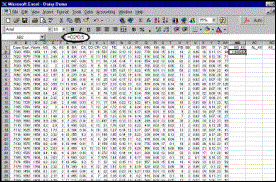Step 1 A new variable, AG_AS is created; this is the square root of AG (suffix _AS is used instead of _S to facilitate sorting later).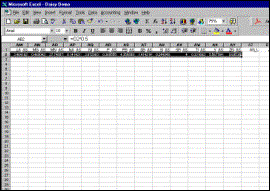Step 2 Square roots of  all input variables are calculated for first sample using “drag and fill” option.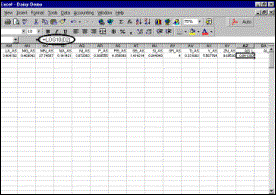Step 3 A new variable, AG_L is created; this is the logarithm (base 10) of AG.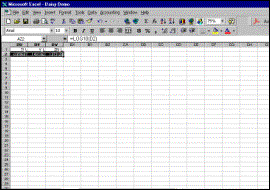Step 4 Logarithms of  all input variables are calculated for first sample using “drag and fill” option.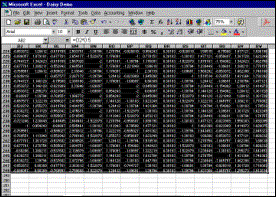Step 5 Square roots and logarithms of all elements on all remaining samples are calculated using “drag and fill” option.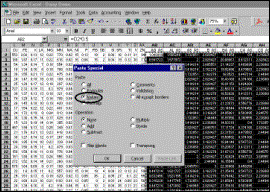Step 6 Calculations are “fixed” using Copy/Paste Special command.Step 7 Data are sorted left to right to bring square-roots and logarithms next to variables from which they were calculated.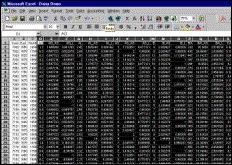Step 8 Result. Suffix “_AS” can be edited to “_S” at this point, if desired.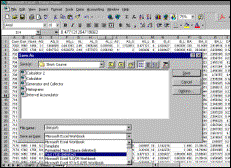Step 9 Output is saved as tab-delimited text file for input to Systat.Step 10 The first of these messages appears when an attempt is made to save the file as text. Click on “OK”. The second appears when the file is closed or when Excel is exited. Click on “No”.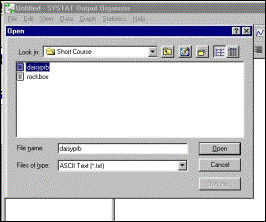Step 11 Open the data file just created in Excel.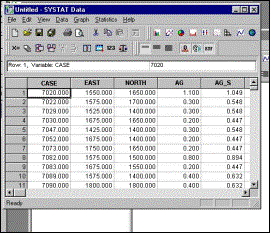Step 12 Data window opens automatically.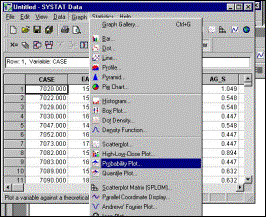Step 13 Select “Probability Plot” from “Graph” menu. Note: Unlike Box Plot, there is no Icon on the standard toolbar for this option.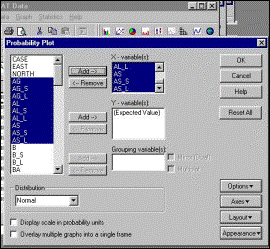Step 14 The untransformed, square-root transformed and log-transformed distributions of each variable should be viewed simultaneously to facilitate comparison. In this case, three elements (Ag, Al and As are being viewed at once).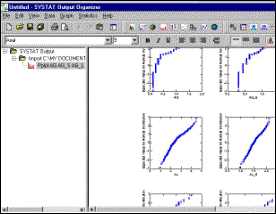Step 15 Preliminary result. Double-clicking on the plots opens the graphics window (next frame).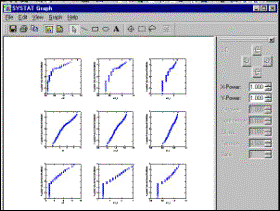Step 16 Ag (first row) approximates to a somewhat censored lognormal distribution (rightmost plot displays straightest line); Al (second row) to a square-root distribution (middle plot displays straightest line) and As (third row) to a square-root distribution, censored almost to 50% (long vertical line, lower left).

Go to Directory Page